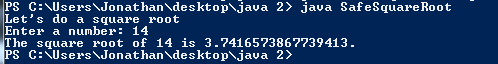# Assignment #74

## Code

```  /// Name: Jonathan Stine
/// Period: 5
/// Program Name: Safe Square Root
/// File Name: SafeSquareRoot.java
/// Date Finished: 5/4/16

import java.util.Scanner;

public class SafeSquareRoot {

public static void main( String[] args ) {

Scanner keyboard = new Scanner(System.in);

int n;

System.out.println( "Let's do a square root" );

System.out.print( "Enter a number: " );
n = keyboard.nextInt();
double sr = Math.sqrt(n);

while ( n <= 0 ) {
System.out.println( "You can't take the square root of a negative number dumbass." );
System.out.print( "Try again: " );
n = keyboard.nextInt();
sr = Math.sqrt(n);
}

System.out.println( "The square root of " + n + " is " + sr + "." );

}
}

```

### Code Output